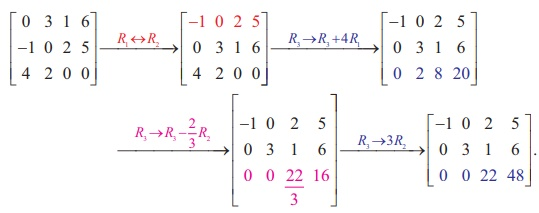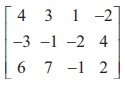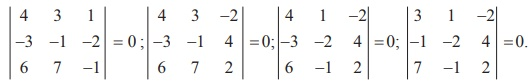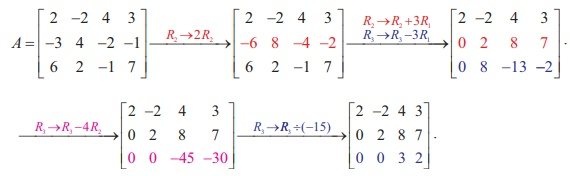Home | | Maths 12th Std | Elementary Transformations of a Matrix: Solved Example Problems

# Elementary Transformations of a Matrix: Solved Example Problems

rank of the matrices by minor method, rank of the matrices by row reduction method, inverse of matrices by Gauss-Jordan method

## Row-Echelon form

### Example 1.13

Reduce the matrixto a row-echelon form.

### SolutionNoteThis is also a row-echelon form of the given matrix.

So, a row-echelon form of a matrix is not necessarily unique.

Example 1.14

Reduce the matrixto a row-echelon form.

Solution## Rank of a Matrix

### Example 1.15

Find the rank of each of the following matrices:Solution

(i) Let A =. Then A is a matrix of order 3├Ś 3. So Žü(A) Ōēż min {3, 3}  = 3. The highest  order of minors of A is 3 . There is only one third order minor of A .

It is=  3 (6ŌłÆ 6) ŌłÆ 2 (6ŌłÆ6) +  5 (3 ŌłÆ 3) = 0. So, Žü(A) < 3.

Next consider the second-order minors of A .

We find that the second order minor= 3 ŌłÆ 2 = 1 ŌēĀ 0 . So Žü(A) = 2 .

(ii) Let A =. Then A is a matrix of order 3├Ś4 . So Žü(A) Ōēż min {3, 4}  = 3.

The highest order of minors of A is 3 . We search for a non-zero third-order minor of A . But

we find that all of them vanish. In fact, we haveSo, Žü(A) < 3. Next, we search for a non-zero second-order minor of A .

We find that= -4+9 =5 ŌēĀ 0 . So, Žü(A) = 2 .

### Remark

Finding the rank of a matrix by searching a highest order non-vanishing minor is quite tedious when the order of the matrix is quite large. There is another easy method for finding the rank of a matrix even if the order of the matrix is quite high. This method is by computing the rank of an equivalent row-echelon form of the matrix. If a matrix is in row-echelon form, then all entries below the leading diagonal (it is the line joining the positions of the diagonal elements a11 , a22 , a33 ,L. of the matrix) are zeros. So, checking whether a minor is zero or not, is quite simple.

### Example 1.16

Find the rank of the following matrices which are in row-echelon form :Solution

(i) Let A =. Then A is a matrix of order 3 3 ├Ś and Žü(A) Ōēż 3

The third order minor |A| == (2) (3)( 1) = 6 ŌēĀ 0 . So, Žü(A) = 3 .

### Example 1.17

Find the rank of the matrixby reducing it to a row-echelon form.

### Solution

Let A =. Applying elementary row operations, we getThe last equivalent matrix is in row-echelon form. It has two non-zero rows. So, Žü (A)= 2.

### Example 1.18

Find the rank of the matrixby reducing it to a row-echelon form.

### Solution

Let A be the matrix. Performing elementary row operations, we getThe last equivalent matrix is in row-echelon form. It has three non-zero rows. So, Žü(A) = 3 .

Elementary row operations on a matrix can be performed by pre-multiplying the given matrix by a special class of matrices called elementary matrices.

### Example 1.19

Show that the matrixis non-singular and reduce it to the identity matrix by elementary row transformations.

### Solution

Let A =.Then, |A| = 3 (0+2 ) ŌĆō 1(2+5) + 4(4-0) = 6-7+16 ŌēĀ 0. So, A is non-singular. Keeping the identity matrix as our goal, we perform the row operations sequentially on A as follows:## Gauss-Jordan Method

### Example 1.20

Find the inverse of the non-singular matrix A =, by Gauss-Jordan method.

### Solution

Applying Gauss-Jordan method, we get### Example 1.21

Find the inverse of A =by Gauss-Jordan method.

### Solution

Applying Gauss-Jordan method, we getTags : with Answers, Solution , 12th Mathematics : UNIT 1 : Applications of Matrices and Determinants
Study Material, Lecturing Notes, Assignment, Reference, Wiki description explanation, brief detail
12th Mathematics : UNIT 1 : Applications of Matrices and Determinants : Elementary Transformations of a Matrix: Solved Example Problems | with Answers, Solution